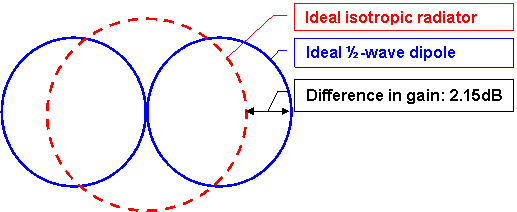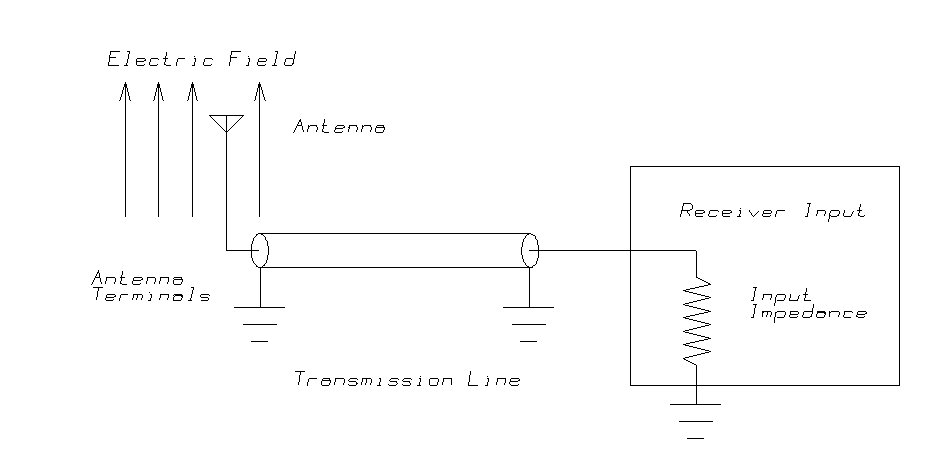Field Intensity Units

Q: What is the difference between dBu, dBm, dBuV, and other units?

A: There is a great deal of confusion when engineers, technicians, and equipment salespersons talk about units of antenna gain and field strength. People in different disciplines of the radio telecommunications industry seem to be speaking different languages and most people are not multi-lingual. This article will discuss units of gain and field intensity and explain how to convert between some of these units when appropriate.

Units of Antenna Gain

While field strength at any location is independent of antenna gain, received voltage at the receiver is not. Therefore, let us first consider antenna gain

Gain may be expressed as either a power multiplier or in dB. Antenna gain stated in dB is referenced to either isotropic or a half-wave dipole. The microwave industry has universally established the convention of reporting antenna gain in dBi (referenced to isotropic). The land mobile industry has almost universally expressed antenna gain as dBd (referenced to a half-wave dipole rather than isotropic.) When a manufacturer lists a gain as dB, you may generally assume that the referenced gain is dBd. Broadcast antenna manufacturers commonly refer to a multiplier gain where the antenna input power is multiplied by this gain to yield the effective radiated power.

The simplest antenna is an isotropic radiator. This is a theoretical antenna that radiates the same level of energy in all directions when power is applied to the antenna. Even though this type of an antenna cannot actually be constructed, the use of the concept provides a uniform standard against which the performance of all manufactured antennas can be calibrated and compared.Figure 1:  Half-wave dipole vs. isotropic antenna

An antenna which can be easily built is a half-wavelength dipole. A half-wavelength dipole antenna has a gain of 2.15 dB greater than an isotropic antenna. The dipole concentrates the energy in certain directions, so that the radiation in those directions is greater than the radiation from an isotropic source with the same input power.

Therefore, the gain of an antenna referenced to an isotropic radiator is the gain referenced to a half-wavelength dipole plus 2.15 dB:

(1) GdBi = GdBd + 2.15

As shown in Figure 1 (and Figure 2) a directional antenna (including a half-wave dipole) can be considered to concentrate the available energy fed into the antenna, focusing the energy radiated from the antenna into the desired direction. The energy radiated in the desired direction(s) is increased by reducing the energy radiated in some other direction(s).

For example, a collinear array of four dipole antennas will typically have a gain of 6 dBd. This same antenna will have a gain of 8.15 dBi (referenced to isotropic).Figure 2: Gain in dBd vs. dBi

Directional antenna patterns are sometimes plotted as gain in dB above a half-wave dipole. Other patterns are shown as a relative field voltage. These are directly transferable as long as one knows the absolute gain in dBd or dBi of the major lobe of the antenna. The equation is as follows:

(2) G(dB) = Gm(dBd) + 20 log Rv

where:

G is the gain in dB on a particular azimuth

Gm is the maximum power gain in dB referenced to a half-wave dipole

Rv is the relative field voltage for the particular azimuth

To convert the gain value (in dB) on a particular azimuth to a relative field value, use the following equation:

(3) Rv = 10(G - Gm)/20

When the maximum effective radiated power and the relative field voltage on a particular azimuth are known, the effective radiated power on that particular azimuth is calculated from the following equation:

(4) Rp = P (Rv)2

where:

Rp is the effective radiated power on a particular azimuth (in watts, kW, etc.)

P is the effective radiated power in the major lobe (max) in the horizontal plane (in watts, kW, etc.)

Units of Field Intensity

There is also a great deal of confusion in the vocabulary for field strength (also called field intensity). Values are commonly expressed in dBu, dBµV, and dBm. Each unit has both merit and common usage in certain disciplines in the radio communications industry. However, the widespread confusion about how they relate to one another causes both frustration and misunderstandings about system design and actual performance. The following terms will be discussed at length.

dBu is E (electric field intensity) always in decibels above one microvolt/meter (dBµV/m)

dBµV (using the Greek letter µ ["mu"] instead of u) is voltage expressed in dB above one microvolt into a specific load impedance; in land mobile and broadcast this is commonly 50 ohms.

dBm is a power level expressed in dB above one milliwatt

Electric Field Intensity

The electric field intensity unit dBu is the unit used extensively by the Federal Communications Commission when referring to field strength. True electric field strength is always expressed in some relative value of volts/meter - never in volts or milliwatts. Electric field intensity is independent of frequency, receiving antenna gain, receiving antenna impedance and receiving transmission line loss. Therefore, this measure can be used as an absolute measure for describing service areas and comparing different transmitting facilities independent of the many variables introduced by different receiver configurations.

When a path has unobstructed line of sight and no obstructions fall within 0.5 of the first Fresnel zone, which would introduce additional attenuation, the received electric field strength will approximate that of free space and may be calculated from the following equation:

(5) E(dBµV/m) = 106.92 + ERP(dBk) - 20 log d(km)

where:

ERP is expressed in dB above 1 kW

d is distance expressed in kilometers

Received Voltage and Power

Although calculations of electric field strength are independent of the receiver characteristics mentioned above, predictions of voltage and received power supplied to the input of a receiver must carefully take each of these factors into account. Correlation between electric field strength and voltage applied to the receiver input is impossible unless all of the above listed information is known and considered in the system design.

When the exact same conditions (path, frequency, effective radiated power, etc.) are applied to identical circumstances, the following equations will allow the system designer to translate between the various systems with complete confidence.

Field strength as a function of received voltage, receiving antenna gain and frequency when applied to an antenna whose impedance is 50 ohms can be expressed as:

(6) E(dBµV/m) = E(dBµV) - Gr(dBi) + 20log f(MHz) - 29.8

Solved for received voltage this equation becomes:

(7) E(dBµV) = E(dBµV/meter) + Gr(dBi) - 20log f(MHz) + 29.8

For Power and Voltage calculations into a 50 ohm load:

(8) P(dBm) = E (dBµV) - 107

Substituting the field value for the voltage from Eq. 7:

(9) P(dBm) = E (dBµV/m) + Gr(dBi) - 20log F(MHz) - 77.2

Note the more general equation for values of impedance (Z) other than 50Ω is:

(8a) P(dBm) = E (dBµV) - 20log(√Z) - 90

And substituting the field value for the voltage from Eq. 7:

(9a) P(dBm) = E (dBµV/m) + Gr(dBi) - 20log F(MHz) - 20log(√Z)  - 60.2

where:

Gr is the isotropic gain of the receiving antenna

Z is the system impedance in Ohms

When a "field strength contour" is plotted and identified in dBm or microvolts (dBµV), it is important to know these values of frequency and antenna gain. The user must understand that such "contours" are only valid for one frequency and the particular receiving antenna gain used for the prediction. There is also a fixed loss in the receiving antenna transmission line - often assumed to be lossless.

For these reasons, such "contours" are ambiguous as coverage predictions, when all receiving antenna gains and transmission line losses are not identical for all receivers. To determine the level of field strength necessary to adequately receive a transmitted signal, use Equation 6 above, taking into account the frequency, receiving antenna gain and required level of receiver voltage for the desired level of quieting in the receiver.

These predictions are for the voltage at the antenna terminals. Actual voltage and power levels at the receiver input must take into account the additional loss present in the receiving transmission line. This loss in signal is particularly critical at high frequencies when cables are long.Figure 3:  Electric field and received voltage and power

Figure 3 summarizes the relationship between electric field strength and the voltage and power at the receiver input terminals.

The electric field strength (in dBu) is a function only of:

• Transmitter effective radiated power.

• Distance from the transmitter.

• Losses from terrain obstructions.

Since the electric field strength is independent of any receiver characteristics, it is a useful standard for computing coverage areas.

The electric field induces a voltage into the antenna, transferring power into the antenna. The voltage (dBµV) at the terminals of the antenna is a function of the gain of the antenna for the particular frequency under consideration. The power (dBm) available at the antenna terminals is also a function of the antenna impedance (usually 50 Ohms).

The transmission line (usually coaxial cable or waveguide) connects the antenna terminals to the receiver input terminals. The voltage and power at the receiver input terminals are reduced by the loss in this transmission line. Transmission line losses are a function of the size and type of the transmission line and the operating frequency. In addition, other losses affect the power transferred to the receiver input terminals. See "Typical Loss Values" in the Technical Reference section for more information about losses inside vehicles, losses due to body proximity with handheld receivers, etc.

Conclusion

The obvious conclusion from this information is that receiving systems with different antenna gains require significantly different electric field strength values for proper operation. A service area contour (in dBµV or dBm) computed for a mobile receiver with a high gain permanently mounted roof antenna can be misleading to users with low gain antenna hand-held units.

Based on the actual equipment proposed and the above equations, the system designer can now calculate the actual field strength necessary for any particular receiving system. Operating the receivers in areas where the field strength meets or exceeds the design level for the equipment can be expected to produce satisfactory system performance. The Field Intensity Grids technical reference section discusses the conversion of electric field intensity values (computed in dBu with TAP) to other units for plotting directly in dBm or dBµV.

Reference material:

Telecommunications Analysis Services User's Reference Guide, Appendix A by Jean Adams, US Department of Commerce, Boulder CO (303) 497-5301

Decibel Products Catalog Number 21, Dallas TX - (214) 631-0310

Electromagnetics, John D. Kraus, Ph.D., McGraw Hill Book Company

"Use Digitized Terrain Data to Plan a Repeater Site", by Michael Wiebe, P.E. and Larry D. Ellis, P.E., Mobile Radio Technology, August 1989I'm interested! Please click here to jump to an online form which helps us better understand your needs. Then we will be able to respond to your request with information that is most useful to you.

Search SoftWright Website

Copyright 1999 by SoftWright LLC